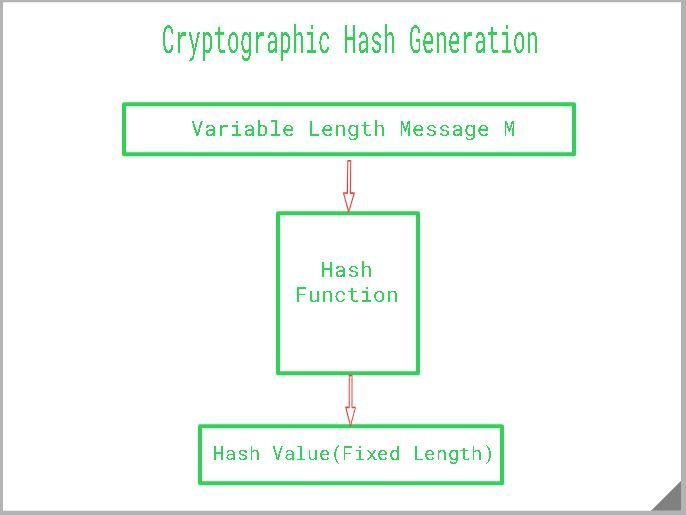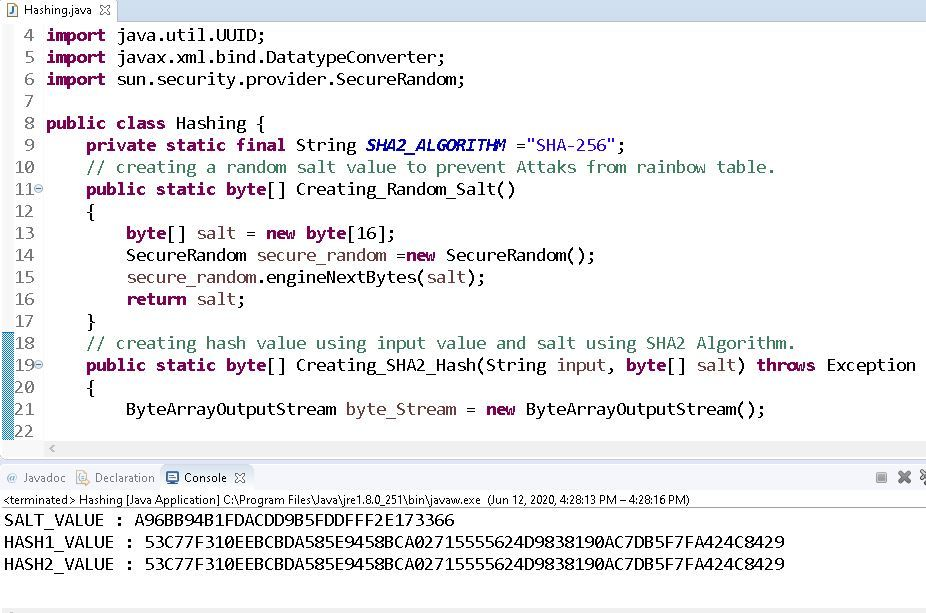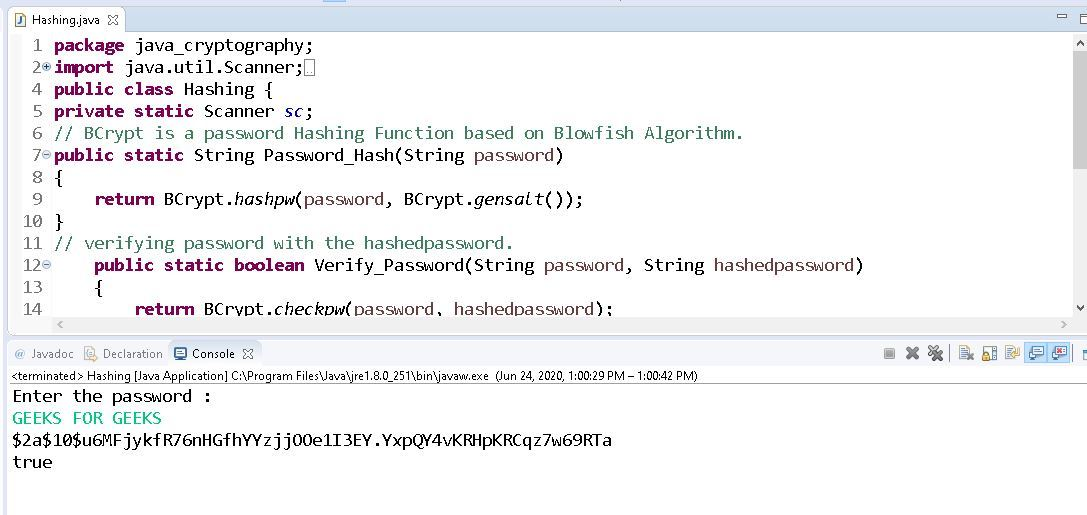Open in App
Not now

# Cryptographic Hash Function in Java

• Difficulty Level : Basic
• Last Updated : 27 Apr, 2022

Cryptographic Hash is a Hash function that takes random size input and yields a fixed-size output. It is easy to calculate but challenging to retrieve original data. It is strong and difficult to duplicate the same hash with unique inputs and is a one-way function so revert is not possible. Hashing is also known by different names such as Digest, Message Digest, Checksum, etc.### Properties Of Cryptography Hash Function

The ideal cryptographic hash function has the following main properties:

1. Deterministic: This means that the same message always results in the same hash.
2. Quick: It is quick to compute the hash value for any given message.
3. Avalanche Effect: This means that every minor change in the message results in a major change in the hash value.
4. One-Way Function: You cannot reverse the cryptographic hash function to get to the data.
5. Collision Resistance: It is infeasible to find two different messages that produce the same hash value.

### Cracking Hash

We often hear the term Cracking a Hash, there are a couple of ways to do that:

• Find an algorithm to generate a collision between two hashes. The more advance the algorithm is, the more difficult it is to crack the hash.
• Another way is to find an algorithm to identify a unique and different input that will produce a given hash. It is similar to a collision, but instead of colliding, we are focusing on finding the input using an algorithm.
• Some common hashes we still use today that are considered “cracked” from a cryptographic point of view are MD5(Message-Digest Algorithm) and SHA-1(Secure Hash Algorithm 1). Keep in mind that these are technically broken Hashes and never use for your security purposes.

### How to create a Cryptographic Hash

• Create a random salt value using SecureRandom class, SecureRandom class generates strong random values. The engineNextBytes(byte[] bytes) method is used to generate a user-specified number of random bytes.
• Convert two sets of bytes into one using ByteArrayOutputStream class and creating it to ByteArray.
• Create an instance of a messageDigest passing SHA2_ALGORITHM which returns a hash of the given input value.
• UUID is used to generate a random number that is converted to string and passed as input.
• The returned object can be converted to a hex binary format using DatatypeConverter.

## Java

 `// Java program to demonstrate` `// how to create a Hash`   `package` `java_cryptography;`   `import` `java.io.ByteArrayOutputStream;` `import` `java.security.MessageDigest;` `import` `java.util.UUID;` `import` `javax.xml.bind.DatatypeConverter;` `import` `sun.security.provider.SecureRandom;`   `public` `class` `Hashing {`   `    ``// Initializing the final string variable` `    ``private` `static` `final` `String SHA2_ALGORITHM` `        ``= ``"SHA-256"``;`   `    ``// Creating a random salt value to prevent` `    ``// attacks from the Rainbow table.` `    ``public` `static` `byte``[] Creating_Random_Salt()` `    ``{` `        ``byte``[] salt = ``new` `byte``[``16``];` `        ``SecureRandom secure_random` `            ``= ``new` `SecureRandom();` `        ``secure_random.engineNextBytes(salt);` `        ``return` `salt;` `    ``}`   `    ``// Creating hash value using input value` `    ``// and salt using the SHA2 Algorithm.` `    ``public` `static` `byte``[] Creating_SHA2_Hash(` `        ``String input, ``byte``[] salt) ``throws` `Exception` `    ``{` `        ``ByteArrayOutputStream byte_Stream` `            ``= ``new` `ByteArrayOutputStream();`   `        ``byte_Stream.write(salt);` `        ``byte_Stream.write(input.getBytes());` `        ``byte``[] valueToHash` `            ``= byte_Stream.toByteArray();` `        ``MessageDigest messageDigest` `            ``= MessageDigest` `                  ``.getInstance(SHA2_ALGORITHM);` `        ``return` `messageDigest` `            ``.digest(valueToHash);` `    ``}`   `    ``public` `static` `void` `main(String args[])` `        ``throws` `Exception` `    ``{`   `        ``// Calling the function Creating_Random_Salt()` `        ``// to generate a random salt value` `        ``byte``[] salt = Creating_Random_Salt();` `        ``System.out.println(` `            ``"SALT_VALUE: "` `            ``+ DatatypeConverter.printHexBinary(salt));` `        ``String valueToHash` `            ``= UUID.randomUUID().toString();`   `        ``// Generating first hash with the salt` `        ``byte``[] hash1` `            ``= Creating_SHA2_Hash(valueToHash, salt);`   `        ``// Generating second hash with exact salt` `        ``// to check if we get the same hash.` `        ``byte``[] hash2` `            ``= Creating_SHA2_Hash(valueToHash, salt);`   `        ``// Print first and the second hash value` `        ``System.out.println(` `            ``"HASH1_VALUE: "` `            ``+ DatatypeConverter` `                  ``.printHexBinary(hash1));` `        ``System.out.println(` `            ``"HASH2_VALUE: "` `            ``+ DatatypeConverter` `                  ``.printHexBinary(hash2));` `    ``}` `}`

Note: Salt is a random value added to the input data(passwords) to defend against pre-computed hash attacks such as Rainbow tables.

Output:

SALT_VALUE: A96BB94B1FDACDD9B5FDDFFF2E173366 HASH1_VALUE: 53C77F310EEBCBDA585E9458BCA02715555624D9838190AC7DB5F7FA424C8429 HASH2_VALUE: 53C77F310EEBCBDA585E9458BCA02715555624D9838190AC7DB5F7FA424C8429### How to create Cryptographic Hashing Passwords

As we have seen how to generate a Hash now, let us use Bcrypt to hash a password. Do not use broken Hashing algorithms for Hashing Passwords. Bcrypt is a password Hashing function based on Blowfish Cipher.

Approach:

• Pass the password to hashpw function which is in Bcrypt class which can also generate the salt by itself and returns a string.
• Verify if the password hash and password are really matching using the checkpw() function. It returns a Boolean value.

Code:

## Java

 `// Java program to demonstrate` `// how to hash a password`   `package` `java_cryptography;`   `import` `java.util.Scanner;` `import` `org.springframework` `    ``.security` `    ``.crypto` `    ``.bcrypt` `    ``.BCrypt;`   `public` `class` `Hashing {`   `    ``// Creating a private instance` `    ``// of Scanner class` `    ``private` `static` `Scanner sc;`   `    ``// BCrypt is a password Hashing` `    ``// Function based on Blowfish` `    ``// Algorithm.` `    ``public` `static` `String Password_Hash(` `        ``String password)` `    ``{` `        ``return` `BCrypt.hashpw(` `            ``password, BCrypt.gensalt());` `    ``}`   `    ``// Verifying password with the` `    ``// hashed password.` `    ``public` `static` `boolean` `Verify_Password(` `        ``String password,` `        ``String hashed_password)` `    ``{` `        ``return` `BCrypt.checkpw(` `            ``password, hashed_password);` `    ``}`   `    ``public` `static` `void` `main(` `        ``String args[]) ``throws` `Exception` `    ``{`   `        ``// Scanner class instance connected` `        ``// to the Input Stream(System.in)` `        ``sc = ``new` `Scanner(System.in);`   `        ``System.out.println(` `            ``"Enter the password: "``);`   `        ``// Scanner class instance` `        ``// reading the user input` `        ``String p = sc.nextLine();`   `        ``// Generate hashed password` `        ``String passwordHash` `            ``= Password_Hash(p);`   `        ``// Print Hashed Password` `        ``System.out.println(` `            ``"Hashed-password: "` `            ``+ passwordHash);`   `        ``// Printing the result of verification` `        ``// of hashed password` `        ``// with original password` `        ``System.out.println(` `            ``"Verification: "` `            ``+ Verify_Password(` `                  ``p, passwordHash));` `    ``}` `}`

Output:

Input: Enter the password: GEEKS FOR GEEKS Output: Hashed-password: \$2a\$10\$u6MFjykfR76nHGfhYYzjjOOe1I3EY.YxpQY4vKRHpKRCqz7w69RTa Verification: true### Hash Uses

• Digital signatures.
• Digital fingerprints.
• Logging sensitive data.